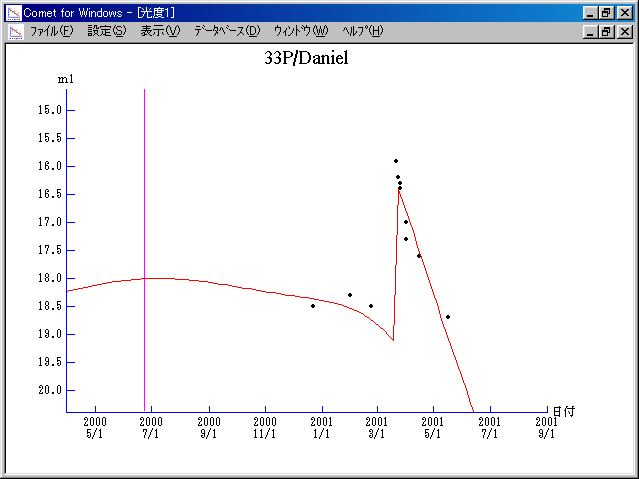# 33P/Daniel (2000)###Orbital Elements

```   The following improved orbital elements by Kenji Muraoka,
are from 60 observations 1979 to 2001, including 9 Planets,
Moon and 5 minor planets perturbations and non-gravitational
effect of style II. The mean residual is +/- 0.88 arc seconds.

Epoch  =  2000 June 25.0  TT       JDT = 2451720.5
T  =  2000 June 23.46617       +/- 0.01221 (m.e.) TT
Peri. =   18.98768                +/- 0.00074
Node  =   66.58265                +/- 0.00017   (2000.0)
Incl. =   22.41004                +/- 0.00011
q  =    2.1572385              +/- 0.0000139 AU
e  =    0.4635952              +/- 0.0000031
a  =    4.0216611              +/- 0.0000087 AU
n  =    0.12220694             +/- 0.00000040
P  =    8.065                  +/- 0.0000262  years
A1  =   +0.295                  +/- 0.025
A2  =   +0.07272                +/- 0.00093
```

###Finding Charts###Magnitudes Graph

```        m1 =  10.5 + 5 log d + 15.0 log r  [   ,260]  (             - 2001 Mar. 10)
m1 = -13.5 + 5 log d + 60   log r  [260,380]  (2001 Mar. 10 - 2001 July  8)
m1 =  10.5 + 5 log d + 15.0 log r  [280,   ]  (2001 July  8 -             )
```##### The orbital elements are calculated by Kenji Muraoka. The charts are made with StellaNavigator Ver.2.0 for Windows (AstroArts / ASCII). The magnitudes graphs are made with Comet for Windows.